# 码农的自我修养——插头DP

Weijie Huang published on
12 min, 2295 words

Categories: Notes Legacy

## 问题背景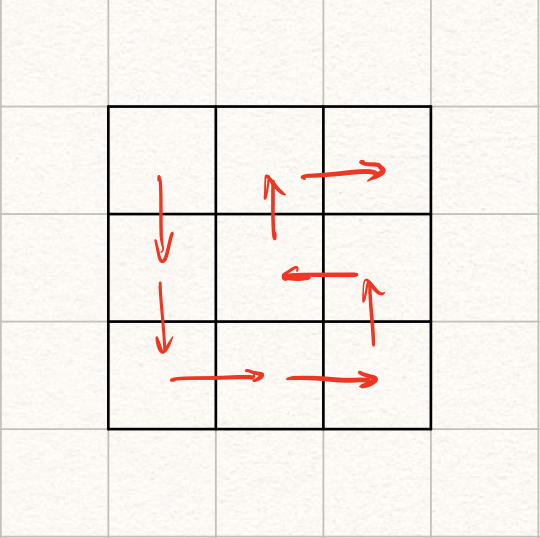## 前置概念

### 插头和括号匹配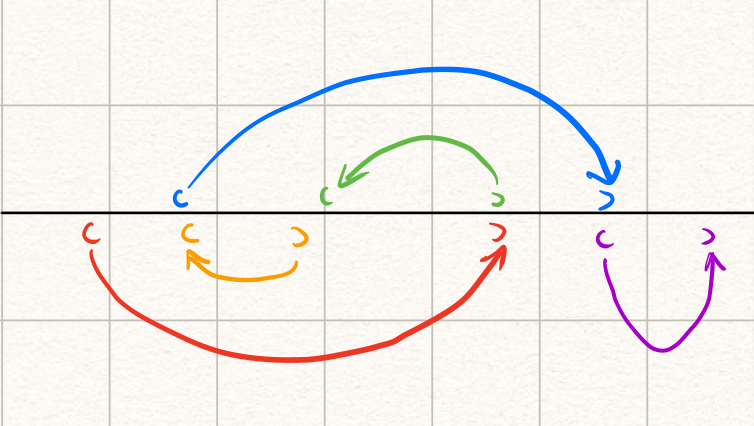### 轮廓线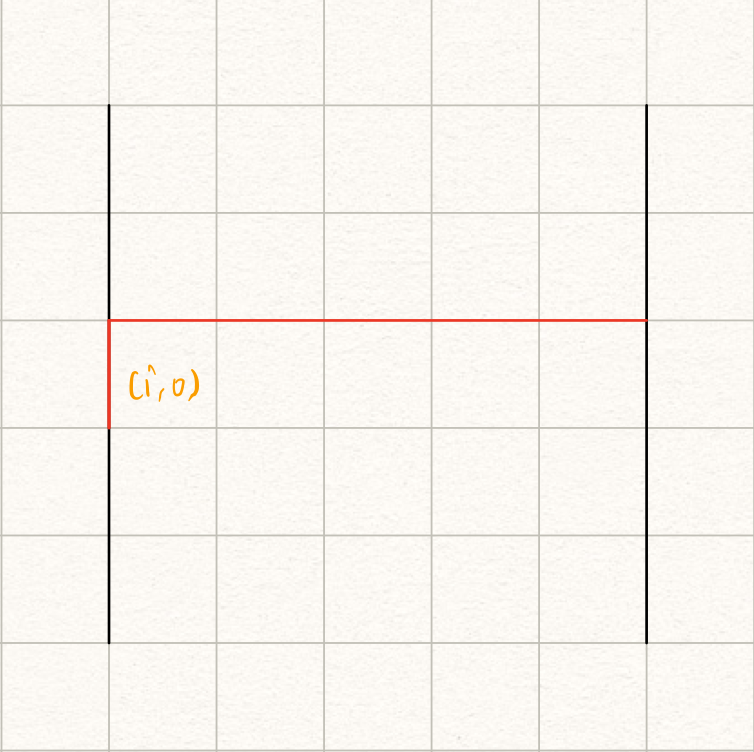## 状态转移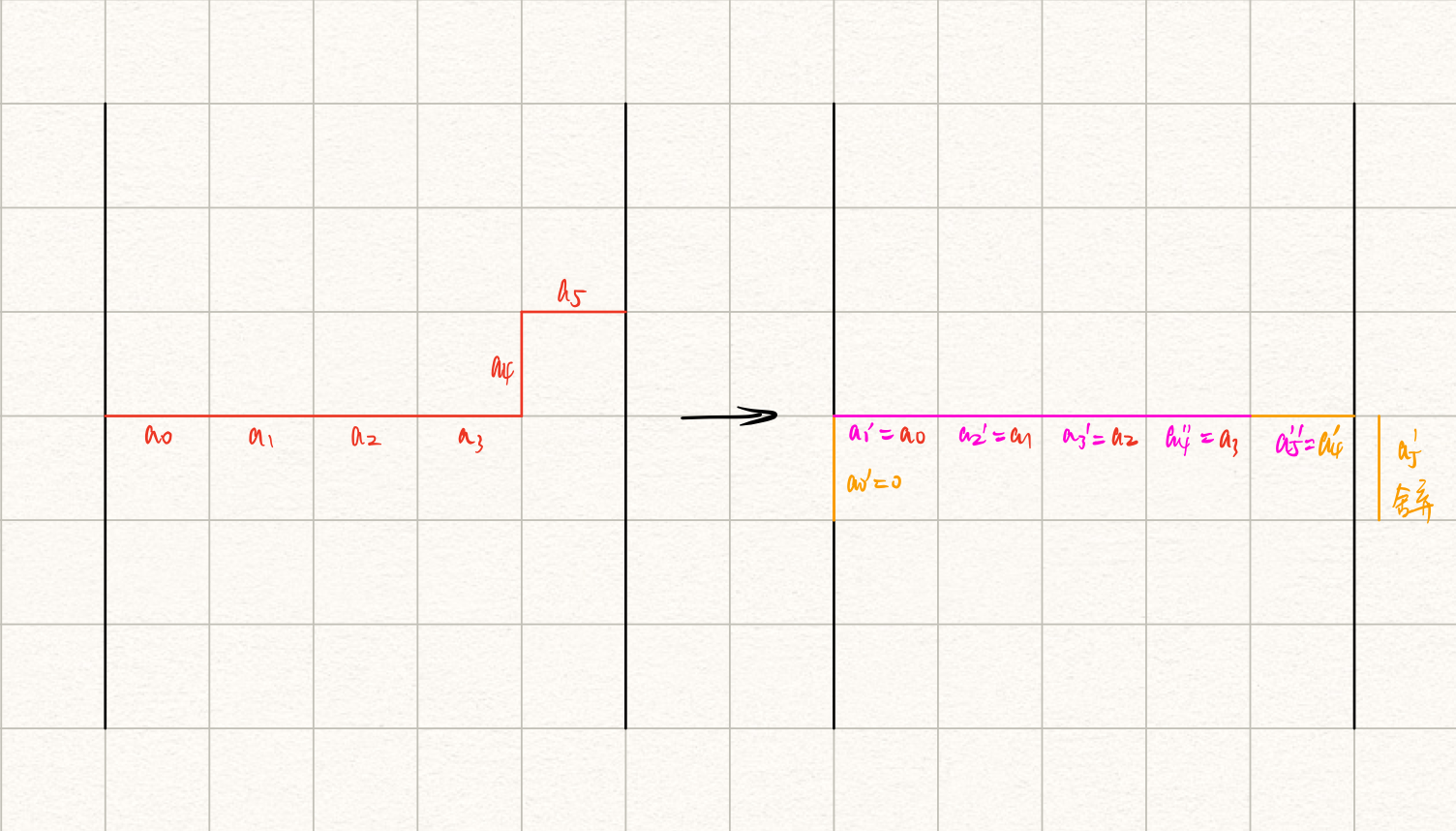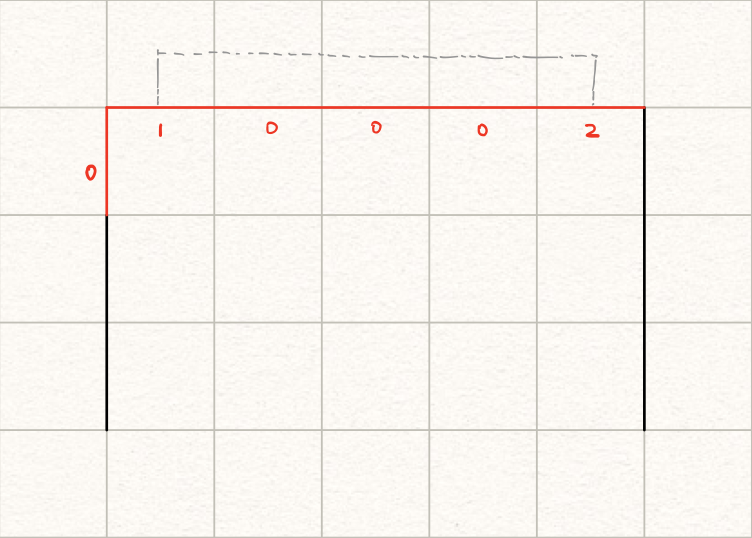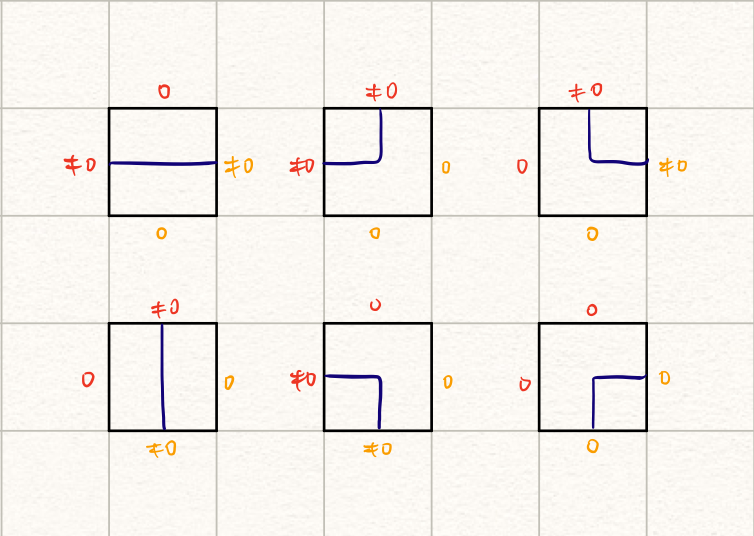1. $(\neq 0, 0)$的情形

只有一个插头的情形，只需要把这个插头（对应的路）拓展一格就好了。我们可以向下做拓展：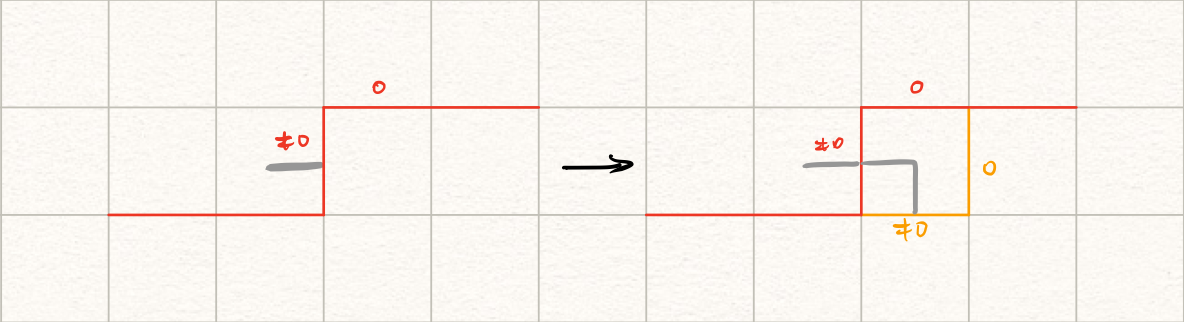注意拓展后的插头类型和拓展前一致。如果当前$j \neq n$，还可以向右做拓展：写成数学符号的形式就是： $$(\neq 0, 0) \rightarrow \begin{cases} (\neq 0, 0)\\ (0, \neq 0) \text{ if } j \neq n \end{cases}$$

2. $(0, \neq 0)$的情形

和上面的情形一样，可以向下或者向右（若$j \neq n$）拓展：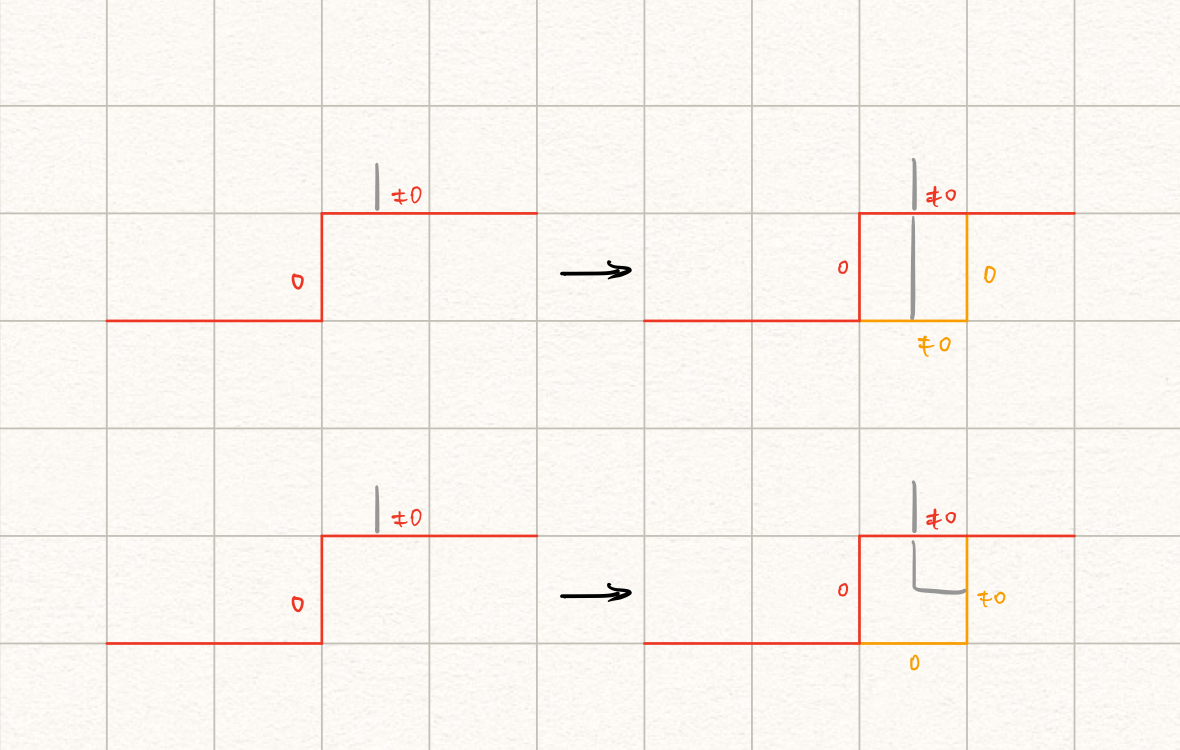用符号表达就是： $$(0, \neq 0) \rightarrow \begin{cases} (\neq 0, 0)\\ (0, \neq 0) \text{ if } j \neq n \end{cases}$$

3. $(0, 0)$的情形

如果两边都没有插头，那就只能 自力更生 新建插头了。为了适应后面的转移，定义新插头状态为$(1, 2)$：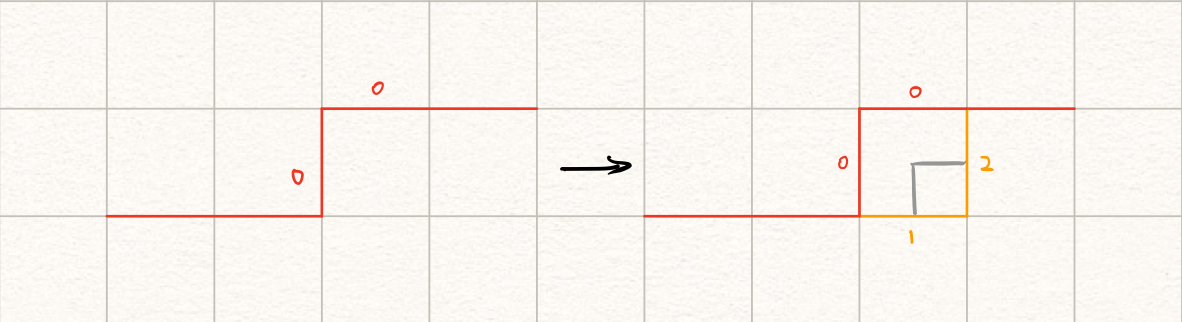符号表达： $$(0, 0) \rightarrow (1, 2) \text{ if } j \neq n$$

4. $(\neq 0, \neq 0)$的情形

两个插头的情形，只要把这两个插头接起来就大功告成了？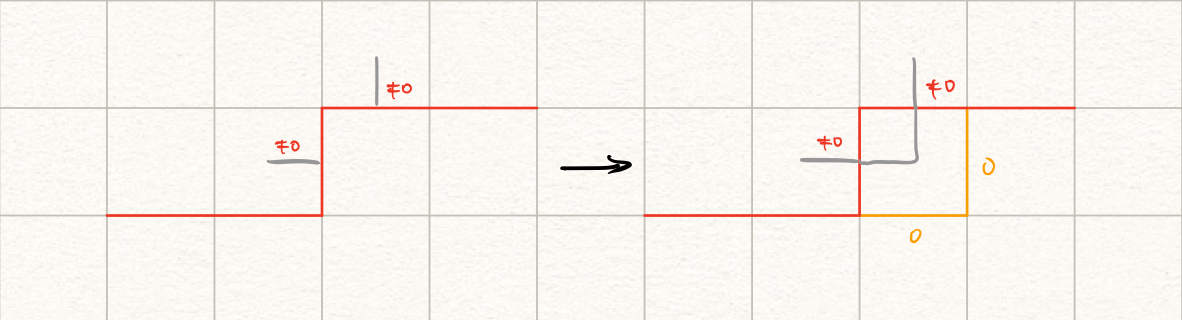符号表达： $$(\neq 0, \neq 0) \rightarrow (0, 0)$$ 错！

对于当前题目，我们需要的是一条Hamilton路，也就是说中间不能出现其它回路。因此我们需要格外注意括号配对的问题。

下面对四种情况进行分类讨论：

• $(2, 1)$的情形

右插头和左插头连接（注意顺序），其实是把两段独立的线接在了一起。没有问题，直接转移。

• $(1, 1)$的情形

左插头和左插头连接，意味着从起点出发的线和一条独立的线接在了一起，作为一条新的起点出发的线。于是我们需要对这条线进行一下修正：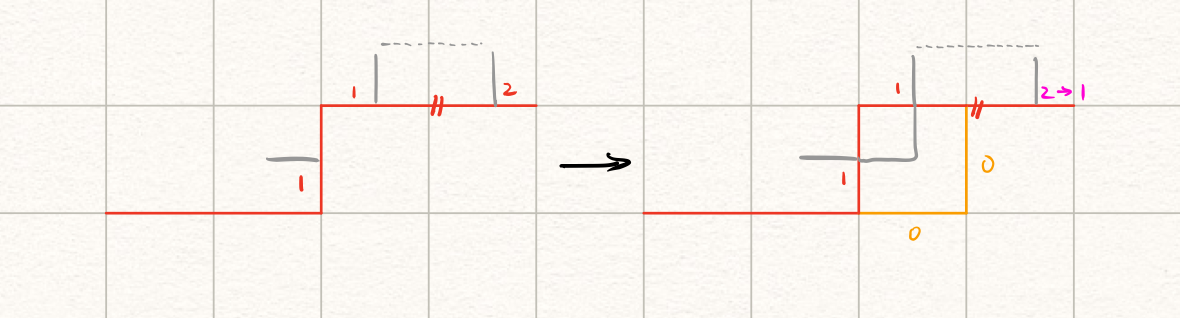如图所示，右边的这个$1$有一个对应的$2$，我们需要将其修改为$1$。我们可以由括号匹配法则向右搜索找到这个$2$。这样一来，整个轮廓线上的括号匹配合法性也得到了保证。

• $(2, 2)$的情形

同理，我们需要对左边的$2$对应的线进行修正。由括号匹配法则向左找到匹配的$1$并修改成$2$。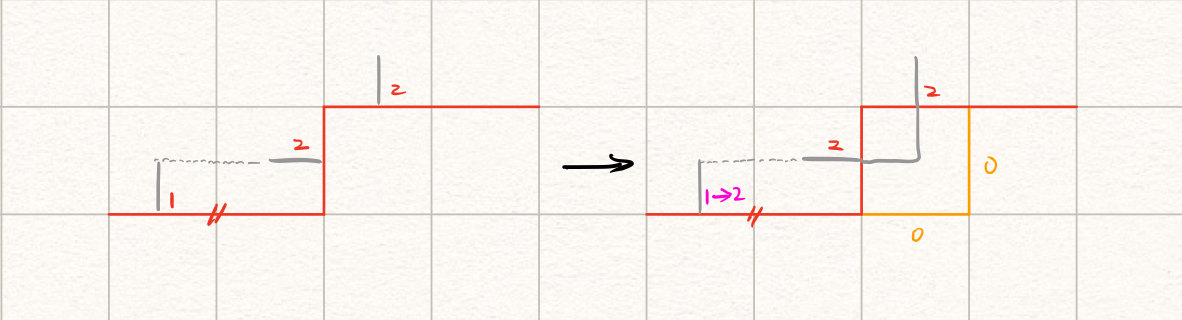• $(1, 2)$的情形

左插头和右插头连接，意味着一个回路的闭合。在这道题目里，我们只能在最后一个遍历到的方格完成这个操作，其余的情况都不能做转移，需要剪枝。

5. 障碍物的情形

障碍物意味着线路需要绕过当前方格，因此也需要状态转移。唯一合法的转移只有$(0, 0) \rightarrow (0, 0)$。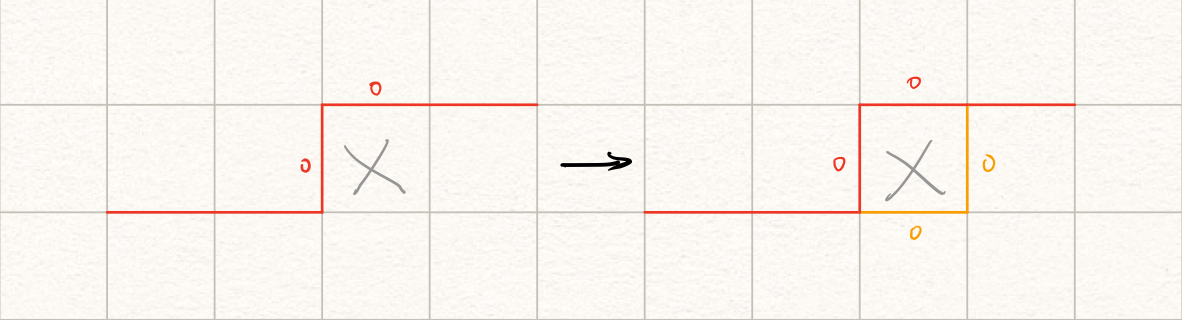## 空间优化

DP一般的做法是使用数组（dp[i][j][S]）来进行转移；然而在这道题里，暴力开数组是会MLE的！